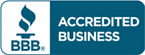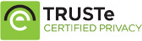# Solution manual for Introduction to Mathematical Thinking Algebra and Number Systems by Will J. Gilbert

\$30.00

Introduction to Mathematical Thinking Algebra and Number Systems by Will J. Gilbert Answers for Questions, exercise and problem sets

Full Chapters are included## Description

Solution manual for Introduction to Mathematical Thinking Algebra and Number Systems by Will J. Gilbert

(NOTE: Each chapter contains exercise and problem sets.)

1. Logic and Proofs.
2. Integers and Diophantine Equations.
3. Congruences.
4. Induction and the Binomial Theorem.
5. Rational and Real Numbers.
6. Functions and Bijections.
7. An Introduction to Cryptography.
8. Complex Numbers.
9. Polynomial Equations.
Appendix: Trigonometry.
Appendix: Inequalities.